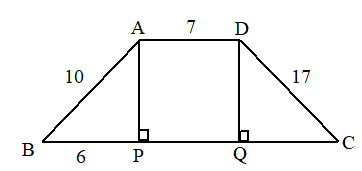# SSC Worksheet for Chapter - 4 Mensuration Class 8

## Find SSC Worksheet for chapter-4 Mensuration class 8

1. CLASS-8
2. BOARD: SSC
3. Mathematic Worksheet - 4
4. TOPIC: Mensuration
5. For otherSSC Worksheet for class 8 Mathematiccheck out main page of Physics Wallah.
1. If are lengths of sides of a triangle, then area
1. 750
2. 650
3. 500
4. 150
2. If the area of an equilateral triangle is , then its altitude is
1. 7√2
2. 9√3
3. 5√6
4. 9√6
3. The perimeter of an isosceles triangle is 42cm and its base is 3/2 times its congruent sides. The area of triangle is
1. 25√2
2. 7√6
3. 27√7
4. 9√5
4. The side of a square play ground is 300m long. Then the cost for leveling the field at the rate of Rs. 1.25/- per square meter is
1. 1,12,500
2. 2,12,800
3. 5,06,890
4. 6,19,200
5. In theABCD figure is a trapezium andABBC . If AB = 10 , BP = 6 , AD = 7, DC = 17 then the area ofABCD is1. 100
2. 120
3. 160
4. 140
6. The perimeter of a semi circle whose radius is 21 units is
1. 108
2. 62
3. 242
4. 42
7. How many rotations does a wheel can whose radius is 35 units, to cover a distance of 2.2km?
1. 10
2. 100
3. 1000
4. 220
8. There are two circles with radii 3 and 4 respectively. Then the radius of circle having area equal to the sum of the areas of these two circles is
1. 5
2. 4
3. 2
4. 7
9. The area of a regular hexagon whose side length is 9 units, is
1. 120.83
2. 296.150
3. 210.438
4. 345.241
10. A 7 sides regular polygon whose each side measures 8cm is inscribed in a circle of radius 6cm. Then the area of regular polygon is
1. 96
2. 892
3. 292
4. 168
11. The sum of the areas of two circles is 770 sq.cm. and radius of one of them is 14cm. Find the radius of other circle.

### Solutions: to worksheet-4 Topic-Mensuration

 1. (a) 2. (b) 3. (c) 4. (a) 5. (d) 6. () 7. (c) 8. (a) 9. (c) 10. (d)